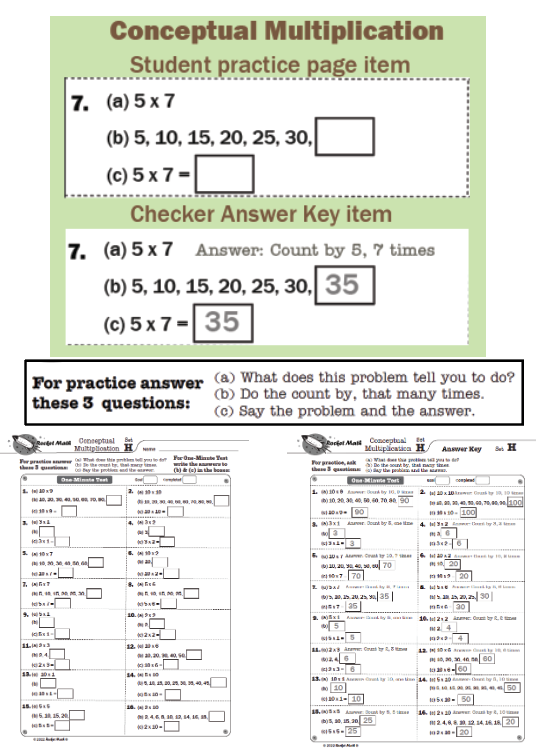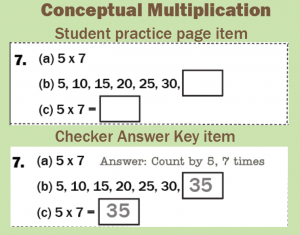## Who needs Conceptual Multiplication?

Students need this learning track only if they have NOT been introduced to the concept of multiplication. This is for students who are not able to figure out the answer to any multiplication fact. When you ask them, for example, “What’s seven times eight?” if they can figure out the answer to that question, then they already understand the concept of multiplication.

## Teaches the concept of multiplication.

Conceptual Multiplication is based upon practicing skip counting or count-bys.  It will be easiest for students after they finish the “Skip Counting” Learning Track. However, in this Learning Track students can read all of the count-by series except the last number, making this somewhat easier than Skip Counting. Students in fourth grade and above (we are in a hurry, as they are late) should be able to do this Learning Track without doing Skip Counting first. Also, students in fourth grade and above (we’re still in a hurry!) do not need to finish all the way to level Z.  They probably have the concept by the time they finish Set H and can go on into the Multiplication (1s-9s) Learning Track.  Just sayin’ we’re in a hurry!

## How do students practice?In each box there are 3 items: a, b, and c.

The checker will only need to read the three questions a couple of times until the student knows what is expected at each letter.

(a)  What does this problem tell you to do? (ex: 5 x 7)    Answer: Count by five, seven times.

(b)  Do the count by, that many times. Answer: 5, 10, 15, 20, 25, 30, 35.

(c) Say the problem and the answer. Answer: Five times seven equals 35.

After a time, the student should just say the answers, for example, “Count by 5 seven times. 5, 10, 15, 20, 25, 30, 35.  Five times seven equals 35.”

For the One-Minute Test students just write the answers in the boxes. In the above example the student would write “35” in both boxes.